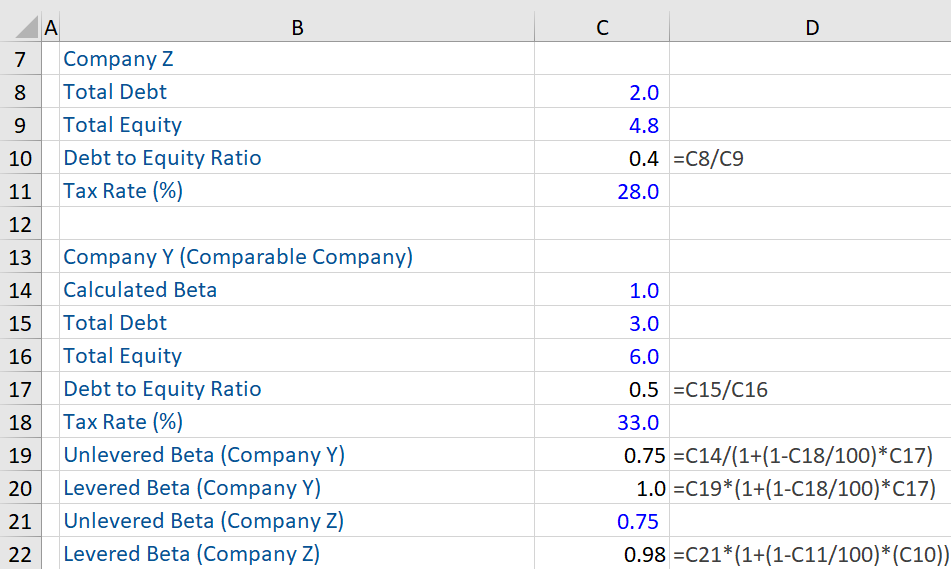## What is “Unlevered Beta”?

Beta (levered beta) is the measure of the systematic risk of a company in relation to the market. The levered beta is a measure of risk that includes the impact of financial leverage. The unlevered beta is the beta of a company, based on the assumption that it uses only equity financing. It measures the market risk of a company without the impact of debt. Unlevering a beta removes the financial impact of leverage and isolates the risk arising solely due to a company’s assets.

Every company has a different capital structure. It is important to measure the riskiness of an individual company’s assets by removing any impact that debt has, and only evaluate how risky the equity of a company is.

## Key Learning Points

• The debt effect must be removed from the levered beta, to calculate the unlevered beta;
• The unlevered beta is lower than the levered beta, as it removes the debt component in the capital structure; and
• When the impact or effect of debt is removed, one can determine the risk of only the company’s assets.

## Unlevered Beta – Formula and Salient Points

The formula to calculate the unlevered beta is:

Unlevered Beta (βa) = Levered Beta (βe)/[1 + ((1-Tax Rate)*(Debt/Equity (D/E) Ratio))]

To calculate the unlevered beta of a company, the debt effect has to be removed from the levered beta – the debt effect can be computed by multiplying the D/E ratio by (1- Tax Rate) and thereafter adding 1 to this value. Once this is done, then we need to divide the levered beta by the debt effect to obtain the unlevered beta.

The unlevered beta is useful when comparing companies with different capital structures, as it focuses on the equity risk. Unlevering the beta does remove the positive or negative impact of adding debt to a company’s capital structure.

The unlevered beta is either equal to or less than the levered beta, since it removes the debt component that adds to the risk. In effect, when a company takes on more debt, it translates to increased risk which is due to increasing leverage rather than market risk. Therefore, by removing the effect of debt, the unlevered beta helps in determining the risk of only the company’s assets.

The unlevered beta of a comparable listed company can be used to create a beta for an unlisted company by re-levering using the unlisted company’s capital structure (see example below). In a similar fashion, you can use the unlevered beta of a more established comparable listed company to create a beta for a recently listed business.

## Unlevered Beta – Example

Assume that Company Z is not a publicly listed company. In such situations, the pure play method is used to calculate the unlevered beta.

Given below is some information on Company Z. To calculate the unlevered beta, we need to search for a company that is comparable to Company Z. This company must be a publicly listed company (Company Y), so that its beta (levered beta) can be computed (which here is 1.0). Assume that Company Y operates in the same sector as Company Z and has a comparable risk profile and product line as this non-listed private company.

We need to calculate the unlevered beta of Company Y (i.e. the effect of leverage has to be removed from the beta of Company Y), which as per the calculation below is 0.75.

Finally, we assume that the unlevered beta of the comparable company (Company Y) is the same as that of Company Z (i.e. 0.75), given that both companies are comparable (as stated above). We have adjusted the unlevered beta of Company Z for its leverage, to get the levered beta value of 0.98.